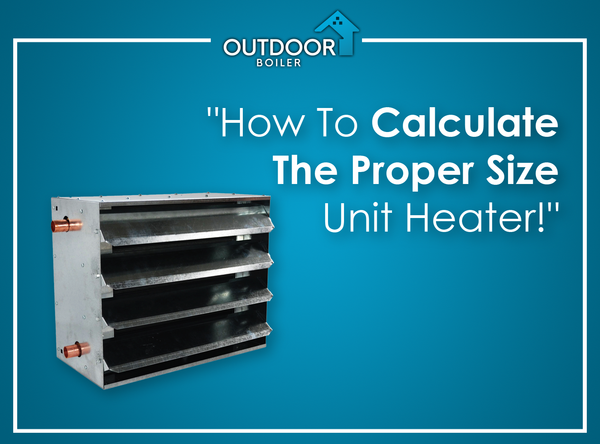# Outdoor Furnace: How To Calculate The Proper Size Unit Heater!If you want to heat a garage, pole barn, a workshop, or any space like that, the best way to do this is with one or more unit heaters. These are very effective at providing heat to large spaces - they contain a blower fan connected to a heat exchanger that allows for heat to be provided directly at the desired location. If desired, the unit heater may be connected to a thermostat for ideal temp control.

To calculate the size of unit heater required for your space, use the following formula:

Calculate the cubic footage of the space to be heated by multiplying building length x width x ceiling height.  A 30 x 40 pole barn with 14-foot average ceilings* will have (30x40x14=) 16,800 cubic feet of space to heat.

Building Length = 30
Building Width = 40
Ceiling Height = 14
30 x 40 x 14 = 16,800 Cubit Feet of Space to Heat

Then multiply the cubic feet times the "Insulation Factor" to get BTUs required to properly heat the space.  Insulation factors are as follows:

4 - Excellent Insulation
5 - Average Insulation
7 - Poor Insulation

So if in this example, let's say you have average insulation and 16,800 cubic feet of space to heat. Multiply 16,800 times 5 equals 84,000. Therefore, you must have one or more unit heaters that produce a total of 84,000 BTUs of heat.

Cubic Feet 16,800 x Insulation Factor 5 = 84,000 BTUs required

Importantly, if you have a space that requires a large amount of heat, you may elect to install more than one unit heater to spread the heat around in the space better. For example, a workshop that needs  250,000 BTUs could be heated with THREE 90,000 BTU unit heaters. Just make sure that the sum of the unit heater BTU output is greater than the calculated BTU requirements.